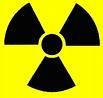# Science Quiz / 'E' & 'F' Units of Measurement

Random Science or Letter Quiz

## Can you name the units of measurement that begin with 'e' and 'f'?

#### byCryptus  Plays Quiz not verified by Sporcle

Score
0/18
Timer
06:00
ClueUnit
A unit of energy or mass in particle physics, defined as the work required to move an electron through a potential difference of one volt.
An old unit of length used primarily for the measure of cloth, equal to 45 inches.
A typographical unit, equal to the currently specified point size e.g. for a 16-point typeface, one of this unit is 16 points.
A unit of time used in astronomy, whereby times are expressed as years and fractions of years.
The unit of work or energy in the CGS system, equivalent to the work done by a force of one dyne acting through a distance of one centimeter.
A unit of temperature.
The SI unit of electrical capacitance.
A unit of length, used today almost exclusively at sea to measure water depth etc.
An alternative name for the femtometre (10-15m), used in atomic physics
An old unit of length, defined as the length of the middle finger, from the tip to the joint where the finger is attached to the hand.
A unit of volume equal to 1/4 of a barrel.
A traditional unit of volume generally equal to the US gallon, or 3.785 litres.
A measure of computing power, equal to one floating point operation per second.
A unit of liquid volume, equal to 1/16 of a pint (US), or 1/20 of a pint (UK).
A unit of length, equal to 12 inches.
A unit of time, equal to 2 weeks.
A measure of the 'speed' of a camera lens; more accurately a measure of the light-gathering power of a lens.
A unit of length, equal to 660 feet or 1/8 of a mile; often used in horse racing.

### You're not logged in!

Compare scores with friends on all Sporcle quizzes.
OR

### Today on Sporcle TV

Watch our educational content, quiz creation guides, and fun videos on Sporcle TV.

### Extras

Created Jan 8, 2016
Tags: